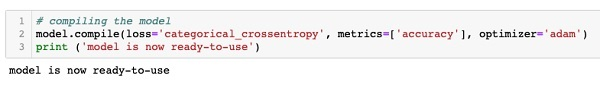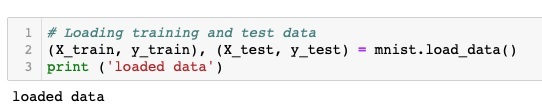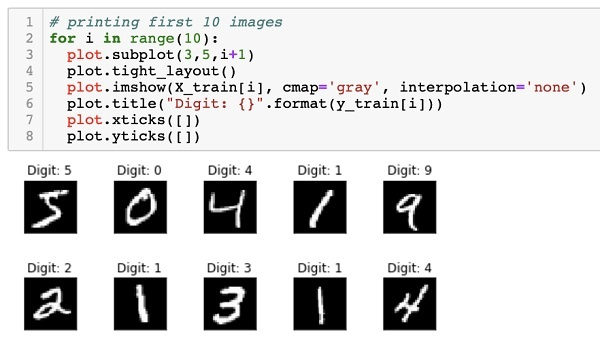# Deep Learning with Keras - Compiling the Model

The compilation is performed using one single method call called compile.

```model.compile(loss='categorical_crossentropy', metrics=['accuracy'], optimizer='adam')
```

The compile method requires several parameters. The loss parameter is specified to have type 'categorical_crossentropy'. The metrics parameter is set to 'accuracy' and finally we use the adam optimizer for training the network. The output at this stage is shown below −Now, we are ready to feed in the data to our network.

As said earlier, we will use the mnist dataset provided by Keras. When we load the data into our system, we will split it in the training and test data. The data is loaded by calling the load_data method as follows −

```(X_train, y_train), (X_test, y_test) = mnist.load_data()
```

The output at this stage looks like the following −Now, we shall learn the structure of the loaded dataset.

The data that is provided to us are the graphic images of size 28 x 28 pixels, each containing a single digit between 0 and 9. We will display the first ten images on the console. The code for doing so is given below −

```# printing first 10 images
for i in range(10):

plot.subplot(3,5,i+1)
plot.tight_layout()
plot.imshow(X_train[i], cmap='gray', interpolation='none')
plot.title("Digit: {}".format(y_train[i]))
plot.xticks([])
plot.yticks([])
```

In an iterative loop of 10 counts, we create a subplot on each iteration and show an image from X_train vector in it. We title each image from the corresponding y_train vector. Note that the y_train vector contains the actual values for the corresponding image in X_train vector. We remove the x and y axes markings by calling the two methods xticks and yticks with null argument. When you run the code, you would see the following output −Next, we will prepare data for feeding it into our network.